# Artificial Intelligence Based Maximum Power Point Tracking Controller for Fuel Cell System

Artificial Intelligence Based Maximum Power Point Tracking Controller for Fuel Cell System
Department of Electrical and Electronics, Mewat Engineering College Nuh, Haryana 122107, India

Corresponding Author Email:
Page:
297-302
|
DOI:
https://doi.org/10.18280/ejee.210306
3 March 2019
|
Accepted:
21 April 2019
|
Published:
15 August 2019
| Citation

OPEN ACCESS

Abstract:

Due to the used of fuel cell (FC) system that produces low pollution, low noise, and high efficiency. Though, an FC's voltage-current response is nonlinear, resulting in only one operating point maximizing the output power given a set of operating conditions. In this paper, a comparative analysis is discussed using a smart controller with the Maximum Power Point Tracking (MPPT) of the Proton Exchange Membrane FC (PEMFC) power system. This paper analyzed the electrical characteristics of PEMFC with regard to the fuel flow rate using FL and conventional perturb and observe (P&O) MPPT techniques. In Matlab/Simulink software and analysis system performance, the proposed system with FL based MPPT controller was modelled under smart control and then optimal power with variable fuel rate. The resulting waveforms were evaluated and demonstrate the system's effectiveness.

Keywords:

PEMFC, dc-dc power converter, MPPT methods, FL controller

1. Introduction

In recent years, the need for renewable energy sources has emerged as a critical issue with growing international concern about the depletion of the world's natural resources and the effects of global warming. Among the numerous available clean energy technologies, such as solar, wind and FC, FC is particularly attractive due to their low pollution, simple maintenance, virtually silent running, long service life and so on. In addition, FC has approximately 40 to 60 percent generating efficiency; with an overall efficiency of more than 80 percent being possible through their integration with cogeneration technologies. As the cost of FC materials continues to decline, the marketing of FC for stationary and standalone applications is increasingly attractive [1, 2]. An FC output voltage depends on the temperature of the cell, the pressure of air and hydrogen, the partial pressure of oxygen and the water content of the membrane. Notably, FC has a characteristic of nonlinear voltage-current (V-I), and thus there is only one single operating point that maximizes output voltage and power under specific operating conditions. Therefore, a requirement of MPPT algorithms that can control stack current and fuel flow to maximize cell efficiency and output power under variable operating conditions .

Several MPPT methods have been described for photovoltaic (PV) systems  and wind turbine (WT) systems , including Hill-climbing like Perturb and Observe (P&O), incremental conductance (INC), short-circuit fractional current (SCFC), open-circuit fractional voltage (OCFV) FL, Neural Network (NN),  current sweep (CS), Ripple correlation control (RCC), drop control of the DC-Link condenser, maximization of current / load voltage and sliding mode control (SMC). Such methods provide an effective way to solve the MPPT problem but vary widely in sensed parameters, complexity, hardware implementation, convergence speed . Different MPPT algorithms were presented to maximize the output power of FC stacks, such as P&O [7-9], adaptive MPPT control [10, 11], motor compressor control , resistance matching , voltage and current based MPPT , and adaptive extreme search (ES) control .

The FC efficiency and output power, as discussed earlier, are highly sensitive to changes in operating conditions. Previous studies suggested reducing the effects of system disturbances on FC performance through sliding mode (SM)  or fuzzy sliding mode (FSM) controllers . In this study, an FC’s output voltage is stabilized at the Maximum Power Point (MPP) using FL based MPPT controller.  Using Matlab / Simulink simulations, the performance of the proposed FC system using FL based MPPT controller is evaluated and compared to P&O based MPPT control systems.

The rest of the research article is structured as follows. description of the proposed system is in Section 2, P&O and FL based MPPT controller are described in Section 3 and Section 4, a simulation model of the complete system is in section 5, results are discussed in Section 6 and conclusions summarized in Section 7.

2. Description of Proposed Pemfc System

This proposed system consists of PEMFC, dc-dc power boost converter, MPPT controllers and load. The block diagram of the proposed system is shown in Figure 1. Optimize the duty cycle with MPPT controllers for achieving maximum power from the FC system. FC system can be used as a backup just like battery backup. Since early 1990, FC has been researched and developed for use in various applications. For examples, portable, backup, transport and stationary power applications.

## 1.png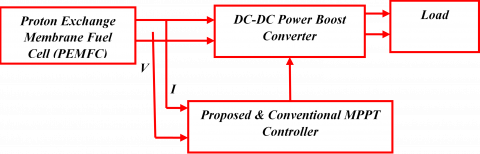Figure 1. Block diagram of proposed PEMFC system

3. P&O Based Mppt Controller

This MPPT method has to measure FC voltage and FC current in the variable atmosphere conditions, calculating the FC power P1 [f], then considering the small changes in the duty cycle and calculating the FC power P2 [f]. If P2 [f] is more than P1 [f], then the direction of perturbation is correct otherwise it should be reversed, the FC power P2 [f] and P1 [f] were compared. It presents disadvantages such as slow response, oscillation about MPP, and even incorrect tracking under rapidly changing atmospheric conditions .

## 22.png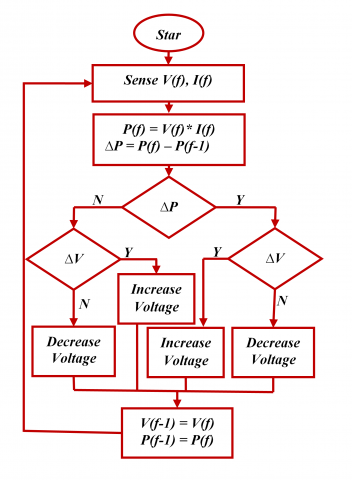Figure 2. Flow chart of P&O algorithm

4. FL Based Mppt Controller

Two input variables are voltage and current of FC stack for the controller. The output of the controller is the duty ratio. These two input variables are converted into fuzzy value using Fuzzification process and then design the fuzzy inference rule based on input parameters. Using the Defuzzification process, the output variable is converted into fuzzy to crisp value. This MPPT method based on fuzzy focuses on switching operation of the dc-dc converter. Figure 3 to Figure 9 shows the details of FL controller .

## 3.png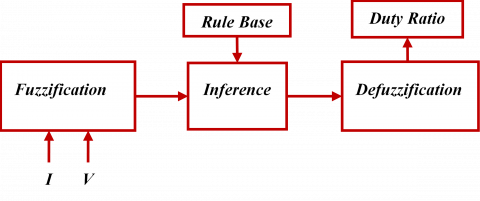Figure 3. Block diagram of FL approach

## 4.pngFigure 4. Fuzzy logic designer

## 6.png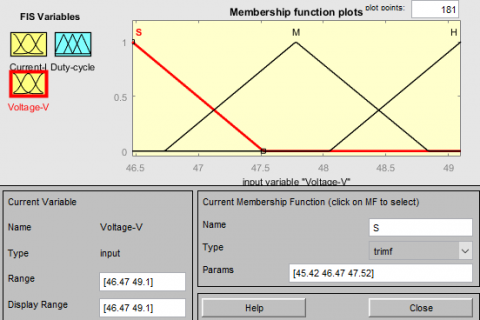Figure 5. Membership function of input (Current-I)

In the design of the FL controller, the main control variables should be identified and the sets describing the values of each linguistic variable and their range should be determined. The input variables are voltage (Voltage-V) and current (Current-I). The calculation and transformation of these input signals into linguistic variables. The output of the FL is the duty cycle (Duty-cycle). The triangular membership functions are used in the intuition method to fuzzify the crisp value into membership value. The interval of the input variable for voltage (46.47 49.1) and current variable (9.708 12.1) and output variable for duty cycle (-0.2 0.8) are appointed. For membership functions, three fuzzy sets are considered and these variables are expressed in terms of the basic fuzzy sets of linguistic variables such as small (S), medium (M) and high (H).

## 6.pngFigure 6. Membership function of input (Voltage-V)

## 7.png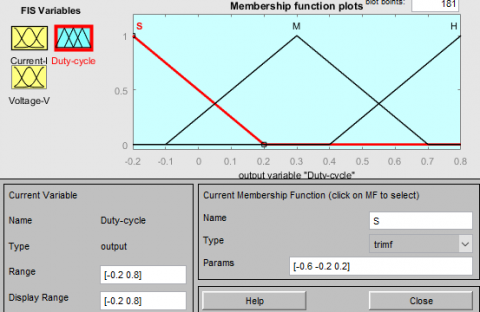Figure 7. Membership function of output (duty-cycle)

## 8.png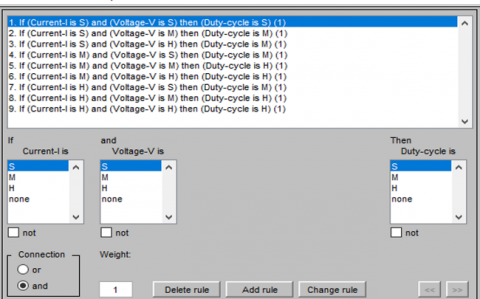Figure 8. Rule editor

## 9.png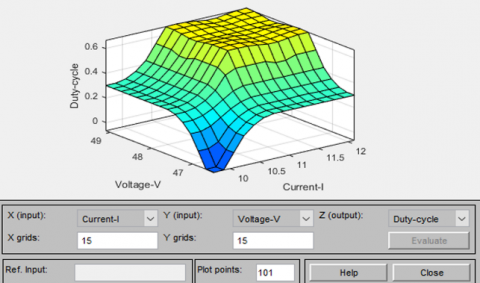Figure 9. Surface viewer of FL controller

## 10.png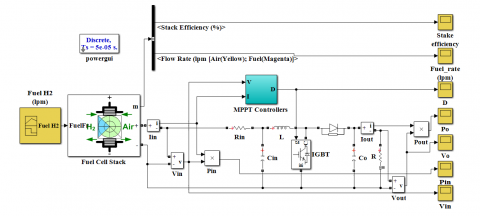Figure 10. Simulation model of FC system with MPPT controller

5. Simulation Model of Complete System

The above-proposed model was simulated using Matlab/Simulink software for simulation and system performance analysis as shown in Figure 10.

To design the simulation model with various MPPT controllers. Parameters are used in the proposed system as given in Table 1. Figure 11 shows the V-I and P-I characteristics of PEMFC system, Figure 12 shows the hydrogen fuel pattern of input for the FC system, Figure 13 and Figure 14 show the Subsystem of P&O controller and FL Controller respectively.

Table 1. Parameter used in proposed PEMFC system

 S. No. Parameter Value 1 Voltage at 0A 65V 2 Voltage at 1A 58V 3 Nominal Operating Point Voltage 28.8V 4 Nominal Operating Point Current 35A 5 Maximum Operating Point Current 45A 6 Nominal and Maximal Power 1008W 7 Resistance of Fuel Cell 0.63Ω 8 One Cell Voltage 1.19V 9 Air Flow Rate 2400lpm 10 Temprature 303K 11 Fuel Flow Rate 31.1lpm 12 Pressure of Supply Fuel 0.5 bar 13 Pressure of Supply Air 1 bar 14 Composition of Fuel (Hydreogen) 99.95% 15 Composition of Oxidant (Oxigen) 21%

## 11.png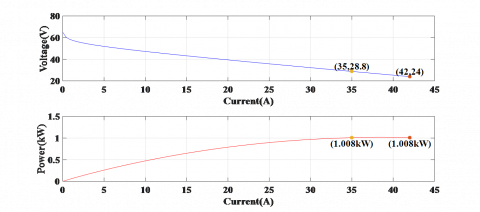Figure 11. V-I and P-I characteristics of PEMFC system

## 12.png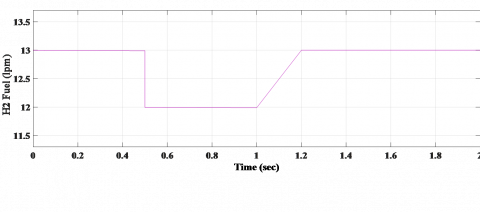Figure 12. Input pattern of hydrogen fuel rate for FC system

## 13.png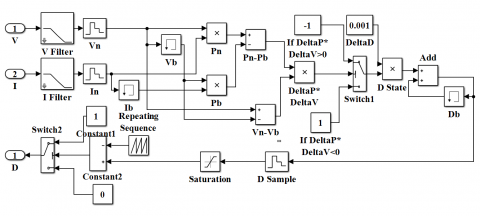Figure 13. Subsystem of P&O controller

## 14.png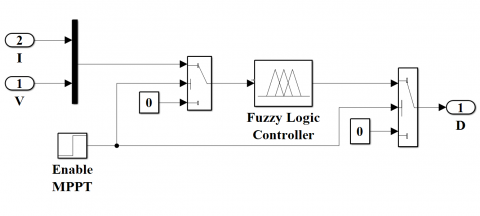Figure 14. Subsystem of FL controller

6. Results and Discussions

In this paper, MATLAB/ SIMULINK™ has been simulated and evaluated.

Figure 15 shows the response of power and voltage before boost converter, it is observed that the approximation value due to more fluctuations of voltage value (in blue) of 88 V and power value (in red) of 500 W, the time taken by MATLAB simulation is 5 s.

## 15.pngFigure 15. Response of power and voltage before boost converter

## 16.pngFigure 16. Output power and voltage after boost converter with P&O MPPT controller

## 17.pngFigure 17. Output power and voltage after boost converter with FL based MPPT controller

## 18.pngFigure 18. Comparative characteristics of output power of boost converter using P&O and FL based MPPT controllers

Figure 16 and Figure 17 show the output power and voltage of boost converter with P&O and FL based MPPT controllers, it is clearly seen that the that the voltage value (in blue) of 200 V and power value (in red) of 400 W using P&O based MPPT controller and the voltage value (in blue) of 210 V and power value (in red) of 450 W using FL based MPPT controller. Similarly, Figure 18 shows the comparative analysis of output power of boost converter using P&O and FL based MPPT controllers. It is clearly observed that the output power value (in red power2) is a greater response as compared to output power value (in blue power1).

7. Conclusions

In this paper, A PEMFC system is stand-alone generation framework consisting of a PEMFC stack, dc-dc power boost converter, MPPT controllers, and the load was developed in MATLAB/ SIMULINK. To discussed PEMFC stack with different MPPT controllers and fuel flow rate, the selecting of the FL controller is best for achieving maximum power from FC system. The FC power system's efficiency has improved from 40 percent to 45 percent using FL controller and demonstrates the effectiveness of the proposed PEMFC system. Also, reduced the fluctuation about the MPP. Future research can be done on economic analysis of the stand-alone as well as on grid-connected PEMFC framework using a hybrid intelligent MPPT controller for different loads.

References

 Hou, Y., Wang, B., Yang, Z. (2011). A method for evaluating the efficiency of PEM fuel cell engine. Applied Energy, 88(4): 1181-1186. https://doi.org/10.1016/j.apenergy.2010.10.040

 Ramos-Paja, C., Romero, A., Giral, R., Calvente, J., Martinez-Salamero, L. (2010). Mathematical analysis of hybrid topologies efficiency for PEM fuel cell power systems design. International Journal of Electrical Power & Energy Systems, 32(9): 1049-1061. https://doi.org/10.1016/j.ijepes.2010.01.032

 Schumacher, J., Gemmar, P., Denne, M., Zedda, M., Stueber, M. (2004). Control of miniature proton exchange membrane fuel cells based on fuzzy logic. Journal of Power Sources, 129(2): 143-151. https://doi.org/10.1016/j.jpowsour.2003.10.010

 Khan, M.J., Mathew, L. (2017). Different kinds of maximum power point tracking control method for photovoltaic systems: A review. Archives of Computational Methods in Engineering, 24(4): 855-867. https://doi.org/10.1007/s11831-016-9192-1

 Khan, M.J., Mathew, L. (2018). Comparative study of optimization techniques for renewable energy system. Archives of Computational Methods in Engineering, pp. 1-10. https://doi.org/10.1007/s11831-018-09306-8

 Esram, T., Chapman,  P.L. (2007). Comparison of photovoltaic array maximum power point tracking techniques. IEEE Transactions on Energy Conversion, 22(2): 439-449. https://doi.org/10.1109/tec.2006.874230

 Giustiniani, A., Petrone, G., Spagnuolo, G., Vitelli, M. (2010). Low-frequency current oscillations and maximum power point tracking in grid-connected fuel-cell-based systems. IEEE Transactions on Industrial Electronics, 57(6): 2042-2053. https://doi.org/10.1109/tie.2009.2034175

 Khanh, L.N., Seo, J.J., Kim, Y.S., Won, D.J. (2010). Power-management strategies for a grid-connected PV-FC hybrid system. IEEE Transactions on Power Delivery, 25: 1874-1882. https://doi.org/10.1109/pesmg.2013.6672094

 Khan, M., Mathew, L. (2019). Comparative study of maximum power point tracking techniques for hybrid renewable energy system. International Journal of Electronics, 106(8): 1216-1228. https://doi.org/10.1080/00207217.2019.1584917

 Zhong, Z.D., Huo, H.B., Zhu, X.J., Cao, G.Y., Ren, Y. (2008). Adaptive maximum power point tracking control of fuel cell power plants. Journal of Power Sources, 176(1): 259-269. https://doi.org/10.1016/j.jpowsour.2007.10.080

 Khan, M.J., Mathew, L. (2018). Comparative analysis of maximum power point tracking controller for wind energy system. International Journal of Electronics, 105(9): 1535-1550. https://doi.org/10.1080/00207217.2018.1461251

 Becherif, M., Hissel, D. (2010). MPPT of a PEMFC based on air supply control of the motocompressor group. International Journal of Hydrogen Energy, 35(22): 12521-12530. https://doi.org/10.1016/j.ijhydene.2010.06.094

 Loo, K., Zhu, G., Lai, Y., Chi, K.T. (2011). Development of a maximum-power-point tracking algorithm for direct methanol fuel cell and its realization in a fuel cell/supercapacitor hybrid energy system. 8th International Conference on Power Electronics-ECCE Asia, 2011, pp. 1753-1760. https://doi.org/10.1109/icpe.2011.5944478

 Sarvi, M., Barati, M. (2010). Voltage and current based MPPT of fuel cells under variable temperature conditions. 45th International Universities Power Engineering Conference UPEC2010, pp. 1-4.

 Bizon, N. (2010). On tracking robustness in adaptive extremum seeking control of the fuel cell power plants. Applied Energy, 87(10): 3115-3130. https://doi.org/10.1016/j.apenergy.2010.04.007

 Abdi, S., Afshar, K., Bigdeli, N., Ahmadi, S. (2012). A novel approach for robust maximum power point tracking of PEM fuel cell generator using sliding mode control approach. International Journal of Electrochemical Science, 7: 4192-4209.

 Zhu, F., Winfield, A., Melhuish,C. (2003). Fuzzy sliding mode control for discrete nonlinear systems. Transactions of China Automation Society, 22.

 Vinod, V., Singh, A. (2018). A comparative analysis of PID and fuzzy logic controller in an autonomous PV-FC microgrid. 2018 International Conference on Control, Power, Communication and Computing Technologies (ICCPCCT), 2018, pp. 381-385. https://doi.org/10.1109/ICCPCCT.2018.8574237